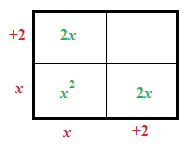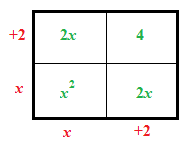### Home > CAAC > Chapter 10 > Lesson 10.3.2 > Problem10-114

10-114.

Use your generalized process of completing the square to rewrite and solve each quadratic equation below.

1. $x^2+4x=-3$

Create a generic rectangle.What number needs to be added to both sides to complete the square?$(x+2)^2=-3+4$

$(x+2)^2=1$

$x=-3$ or $-1$

1. $x^2-8x+7=0$

Subtract $7$ from both sides.

Follow the steps in part a.

$(x-4)^2=9$

$x=1$ or $7$

1. $x^2-24x+129=0$

Follow the steps in part (b).